# AP Statistics : How to estimate margins of errors

## Example Questions

### Example Question #1 : Estimation

The next local election is predicted to have a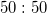split in votes for the top two candidates. How many people should be polled to obtain a margin of error of 3% at the 95% confidence level?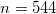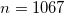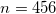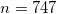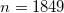Explanation:

If you remember the required formula, this problem is rather simple. Plug in the given numbers and simplify: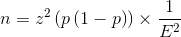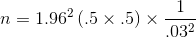If you don't remember the formula, this problem is more challenging. Your best bet in this case is to construct the confidence interval and rearrange to solve for the required sample size.

### Example Question #2 : How To Estimate Margins Of Errors

When dealing with confidence intervals, the margin of error gets smaller when z* gets ________ and n gets _________.

smaller . . . smaller

There is no way to affect the margin of error in confidence intervals

larger . . . larger

smaller . . . larger

larger . . . smaller